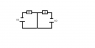# Very simple circuit of 2 batteries

aaaa202
I have some trouble understanding the equations that you get from Kirchoffs law when there are several batteries in a circuit. Consider a circuit with two batteries like the attached.
Kirchoffs laws just gives ohms law for the two circuits as were they completely independent:
U1 = RI1
U2 = RI2

But how does this make sense. I always understood Kirchoffs laws as a rule developed by the basic idea that current flows such that no charge piles up anywhere. Can anyone explain why the above exactly insures this property? Why can none of the current from one battery flow through the other?

#### Attachments

•circuit.png
745 bytes · Views: 374

voko
The semicircuit on the left can be regarded as connected in parallel with the conductor in the middle. So the current from the battery on the right flows in parallel through the conductor and the other battery. Now, with parallel connections, currents are inversely proportional to resistances. The resistance of the conductor in the middle is zero, so the current through it is infinitely greater than the current through the other battery. Which means the latter is zero.

aaaa202
okay thanks. That cleared it up a bit. But what if there was also a resistance in the wire between the two batteries. When doing Kirchoffs laws you would get:
U1 = R1I1 + (I1+I2)Rc
U2 = R2I2 + (I1+I2)Rc

Why does it make sense that the total potential drop for either of the batteries also takes into account the potential drop of the current from the other battery (e.g. for battery 1 you have a term I2Rc), when clearly that current "belongs" to the other battery.
And why do you not have to assume that some of the current I1 could go through battery 2?

#### Attachments

voko
Imagine Rc is very large - infinite, which means there is an air gap there. Do you think no current at all would flow through the circuit?

NoPoke
Both your examples are linear circuits. These have the wonderful property that just like 1+1 = 2 the circuit can be considered as a superposition of simpler systems.

You can turn all batteries off bar one and work out the result for that battery. Then by proceeding in turn with a different battery active get another result. When you have node voltages for all the batteries you simply add them together!

aaaa202
Okay. But saying that doesn't really give an explanation as to why the circuit has that property. What is it physically that enables us to treat the circuit in that way?

NoPoke
Superposition is a property of linear systems. The why is because the system is linear.

Take any linear circuit of batteries and resistors. If you double all the batteries then all the voltages and currents double too. What is less obvious but still true is that if you just vary one of the batteries then the response at all the other points in the circuit is proportional to the change in voltage of that battery. If this was not true then the first example of doubling every battery producing a doubling of every voltage would not work.

----

So the answer to your question of Why can none of the current from one battery flow through the other? Is that it CAN! Just imagine what happens as one of the batteries runs down: at some point the other batteries in the circuit will start to push current into the weakening battery.

Last edited:
aaaa202
But should we not then try split I1 into the part which goes through the middle resistor, and the part which goes through the other battery?

NoPoke
yes and no. you can split the current however you like but the circuit is still going to sort them all out to give the same answer. Again this is a property of linear systems and is just like the additions: 1+1 = 2 and 3 - 1 = 2

So "yes" you can draw the current loops however you wish, "no" because the result will be the same no matter how you draw the loops. You can draw a figure of eight if you want but you may find that some of the paths cancel. In the end you learn to draw convenient loops that yield the unknowns directly or follow a convention such as clockwise only to make checking your work simpler.

voko
In the middle top junction, you have three unknown currents. The three resistances have three unknown voltages. KCL + KVL + Ohm's law will give you 6 linear equations. In the matrix form, they are Ax = b, with the given voltages in b. Now, if b = c + d, and Ay = c and Az = d, then (Ay + Az) = A(y + z) = (c + d) = b, so x = y + z is a solution. c and d may be selected so that only one given voltage is non-zero. That's why you can "switch off" power sources and add solutions linearly. Note, however, that "switch-off" means that you replace the source with a conductor, NOT break the circuit there.